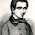## Friday, June 1, 2018

### How mathematicians connected with physicists

ProfessorDavid R. Morrison just posted Geometry and Physics: An Overview:
We present some episodes from the history of interactions between geometry and physics over the past century.
He says "we", but he is the sole author.
In 1954, during the era of minimal communication between mathematics and theoretical physics, C. N. Yang and R. L. Mills [YM54] introduced gauge transformations consisting of locally varying symmetries taking values in a compact Lie group3 G, and studied physical theories which are invariant under such gauge transformations. These generalized the already-familiar abelian gauge transformations from electromagnetism - the same ones we encountered in Section 1 - for which G = U(1). These gauge theories (or "Yang-Mills theories") eventually became the basis of the Standard Model of particle physics, the formulation of which was finalized in the mid 1970s using the group4 G = (SU(3) x SU(2) x U(1))/Z6.

In the late 1960s and early 1970s, Yang got acquainted with James Simons, then the mathematics department chair at SUNY Stony Brook where Yang was a professor of physics. In the course of their conversations,5 Yang and Simons came to recognize that there were important similarities between formulas which were showing up in Yang's work, and formulas which appeared in parts of mathematics which Simons was familiar with. Simons identified the relevant mathematics as the mathematical theory of connections on fiber bundles, and recommended that Yang consult Steenrod's foundational book on the subject [Ste51] (which coincidentally was published just a few years prior to the work of Yang and Mills). Yang found the book difficult to read, but through further discussions with Simons and other mathematicians (including S.-S. Chern) he came to appreciate the power of the mathematical tools which fiber bundle theory offered. ...

Simons communicated these newly uncovered connections with physics to Isadore Singer at MIT who in turn discussed them with Michael Atiyah of Cambridge University. It is likely that similar observations were made independently by others.
I have heard this story directly from Singer, Chern, and others, but I find it hard to believe.

I believe Chern said that Yang took a differential geometry course from him in China, before that 1954 paper was written. So Yang did not really re-invent gauge theories. Yang was also an ego-maniac, so maybe he pretended to.

Indeed, the names "gauge theory" and "gauge transformation" date back to some mathematical physics by Hermann Weyl in 1918. Wikipedia says that Pauli popularized the first widely accepted gauge theory in 1941. Some of the main ideas seem to have been published as early as 1914.

At some point it must have been obvious that special relativity could be elegantly described as spacetime with the metric dx2 + dy2 + dz2 - dt2, with electromagnetism being a connection on a circle (S1) bundle. But I cannot find who explicitly said this first.

Weyl was very close to saying this in 1919, and so was Kaluza, also in 1919. So the idea that this formulation was only discovered in the 1970s is absurd.

One possible explanation is that mathematicians did not realize the importance of bundles, not derived from tangent spaces, until the 1950s. Also, mathematicians quit talking to physicists around 1950. So mathematicians had an intuitive understanding in the 1920s, but would not have expressed it in terms of bundles until the 1950s, and physicists never learned it. I am not sure it ever made it into physics textbooks until recently.

At any rate, the standard model of particle physics is based on replacing the circle with SU(3)xSU(2)xU(1), and using the same geometric formalism. So you would think that physicists would think that the geometric interpretation of electromagnetism would be fundamental and important enough for elementary textbooks.

While Morrison's paper has many examples of mathematical advances related to geometry and physics, the gauge theory of the standard model is the only one that involves genuine physics. The others could be more accurately described as mathematics that was partially inspired by physics, but which does not actually apply to any physical situation.

#### 1 comment:

1.Call me arrogant but isn't it rather easy to reinvent such gauge theories? See this class where the intersection of analysis, algebra and geometry (differential geometry) looks like the third eye of mickey mouse. Ahhh physics... when my brain needs some easy thoughts.